Ex 10.4

Chapter 10 Class 9 Circles
Serial order wise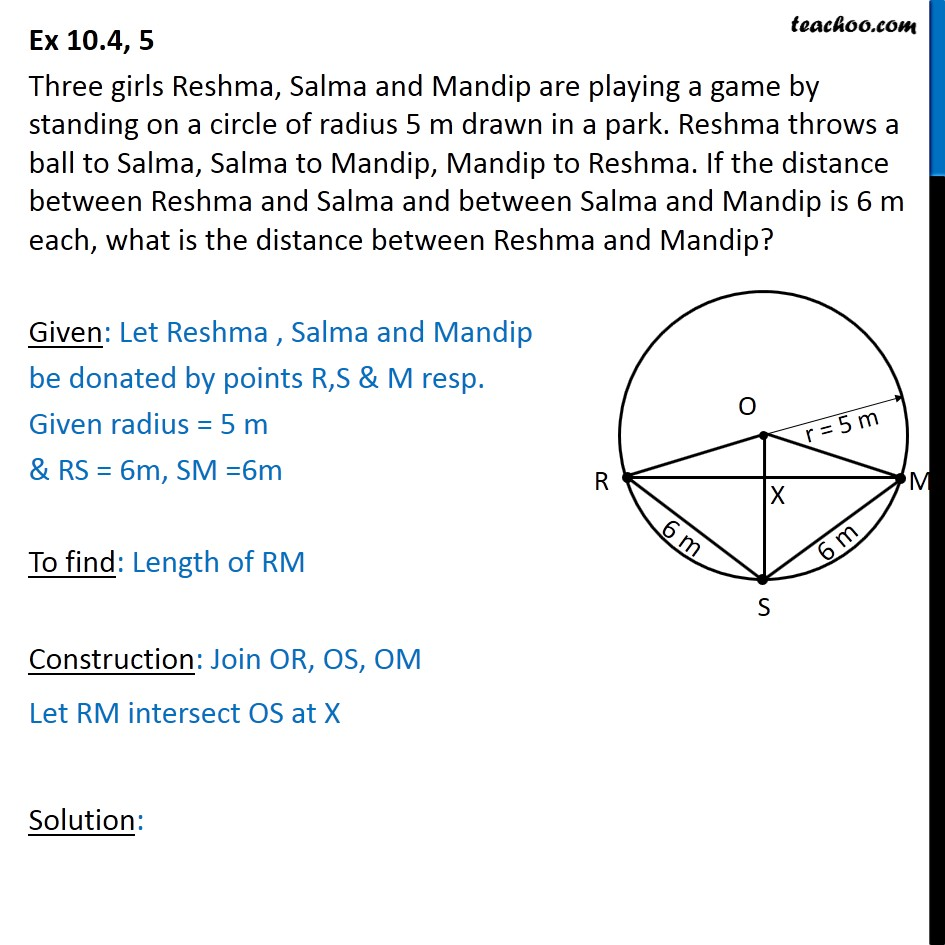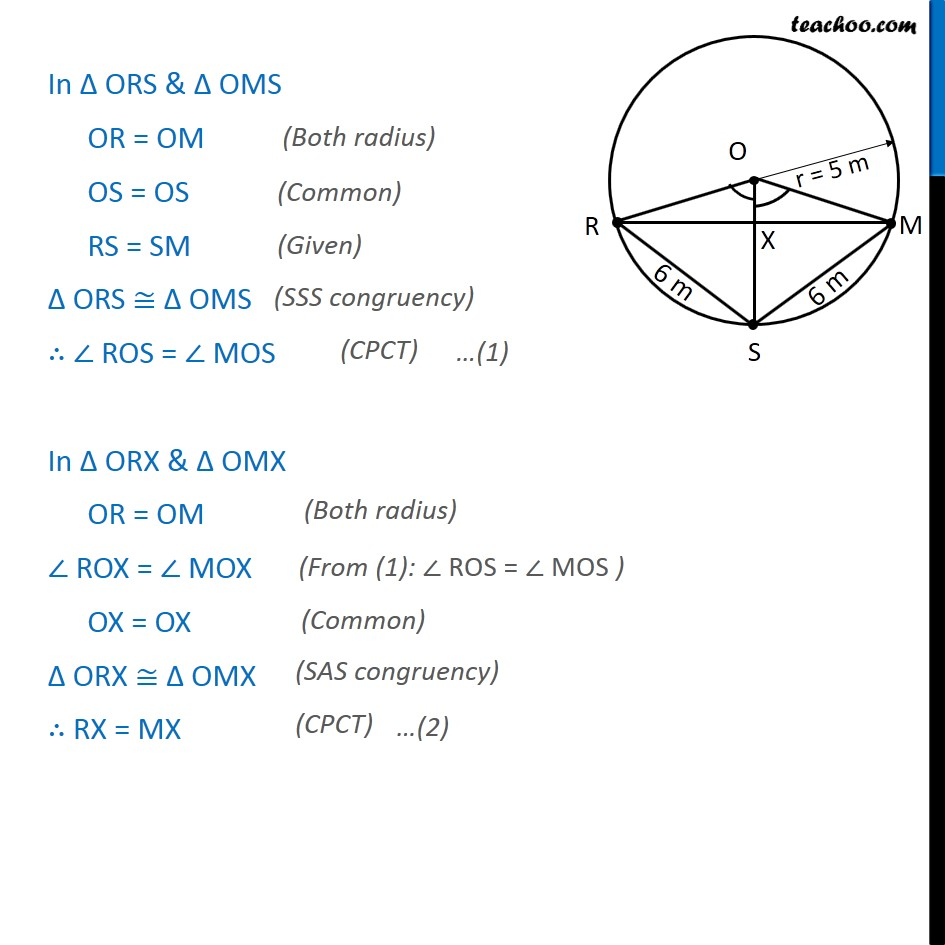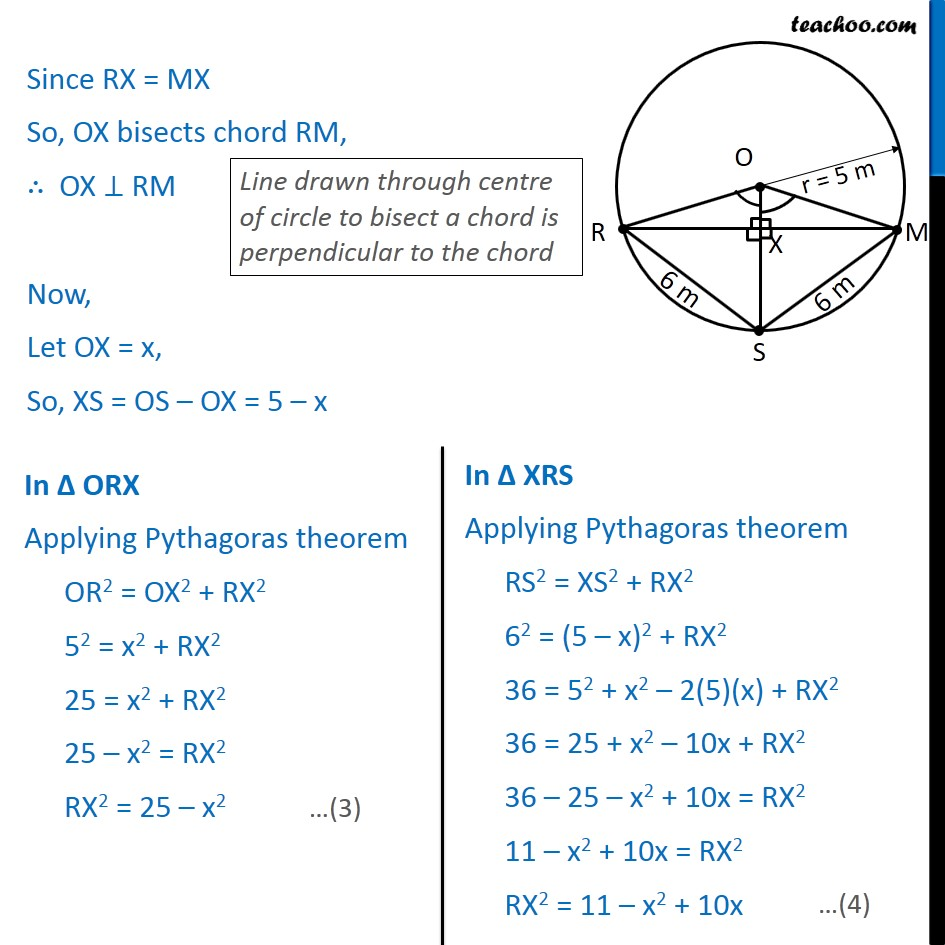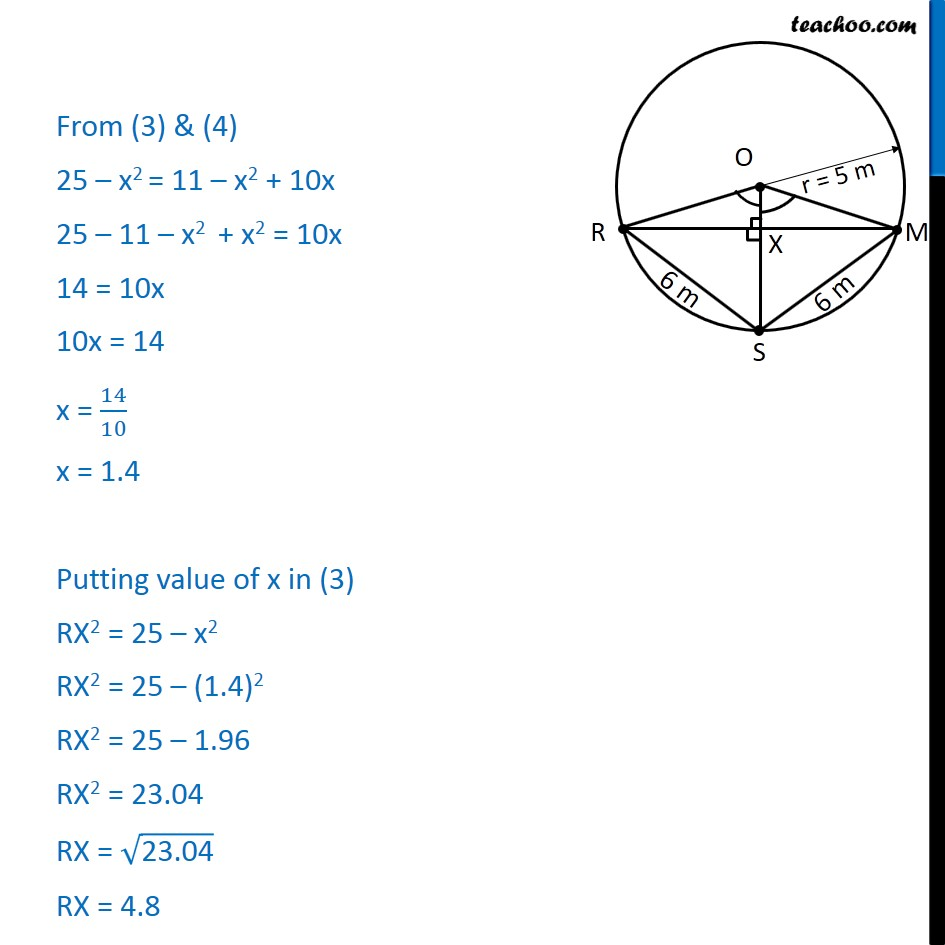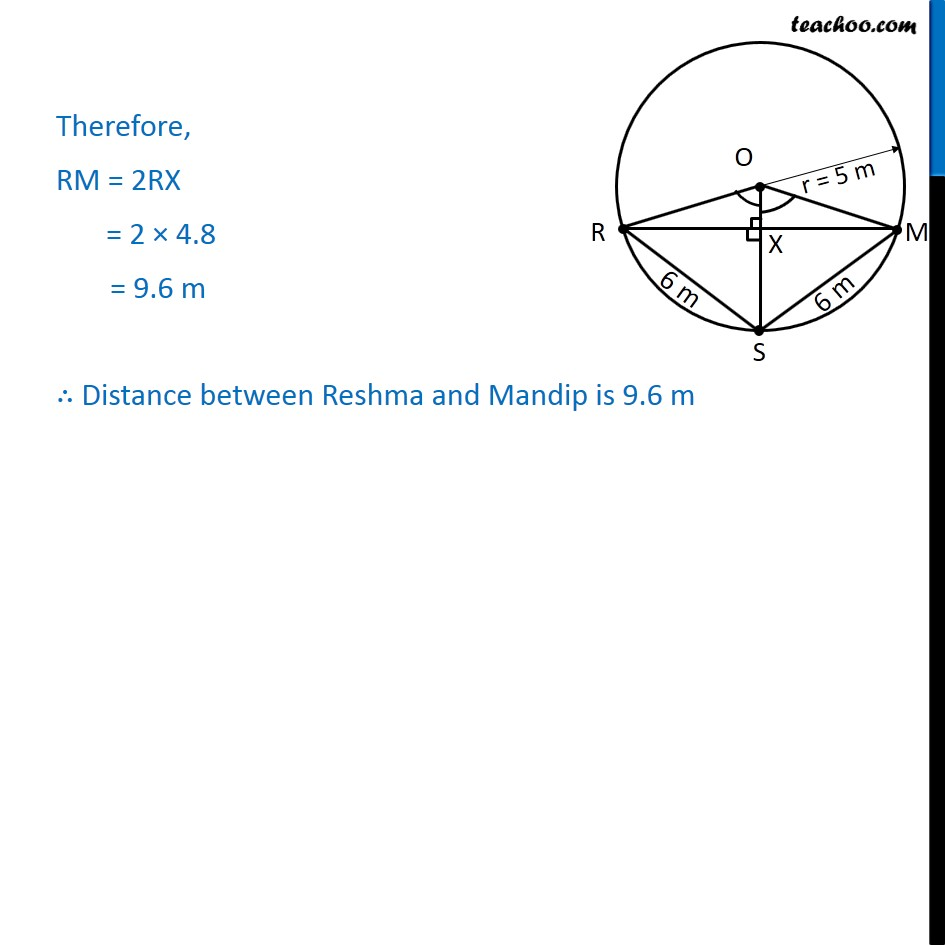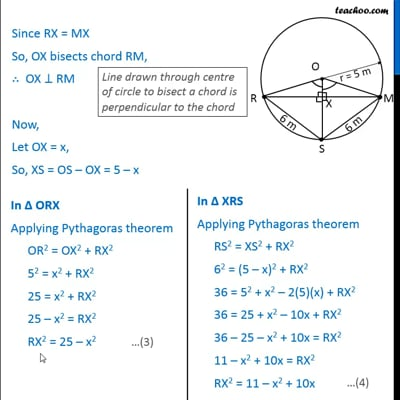This video is only available for Teachoo black users

Solve all your doubts with Teachoo Black (new monthly pack available now!)

### Transcript

Ex 10.4, 5 Three girls Reshma, Salma and Mandip are playing a game by standing on a circle of radius 5 m drawn in a park. Reshma throws a ball to Salma, Salma to Mandip, Mandip to Reshma. If the distance between Reshma and Salma and between Salma and Mandip is 6 m each, what is the distance between Reshma and Mandip? Given: Let Reshma , Salma and Mandip be donated by points R,S & M resp. Given radius = 5 m & RS = 6m, SM =6m To find: Length of RM Construction: Join OR, OS, OM Let RM intersect OS at X Solution: In ORS & OMS OR = OM OS = OS RS = SM ORS OMS ROS = MOS In ORX & OMX OR = OM ROX = MOX OX = OX ORX OMX RX = MX Since RX = MX So, OX bisects chord RM, OX RM Now, Let OX = x, So, XS = OS OX = 5 x From (3) & (4) 25 x2 = 11 x2 + 10x 25 11 x2 + x2 = 10x 14 = 10x 10x = 14 x = 14/10 x = 1.4 Putting value of x in (3) RX2 = 25 x2 RX2 = 25 (1.4)2 RX2 = 25 1.96 RX2 = 23.04 RX = ("23.04" ) RX = 4.8 Therefore, RM = 2RX = 2 4.8 = 9.6 m Distance between Reshma and Mandip is 9.6 m